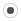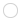unsupported AutoDiff 2nd derivative of sin() etcralf.denzer
Registered MemberPosts
6
Karma
0
OSunsupported AutoDiff 2nd derivative of sin() etc

Sun Jul 28, 2013 11:54 am
Hello,

I came across an error in the unsupported AutoDiff module while using it
to compute the second derivative (the Hessian) of a function.
I like to cite http://forum.kde.org/viewtopic.php?f=74&t=110376
where alexanderwerner and Gael gave me the hints, how to
compute the second derivative.
The second derivative works perfect in the case of polynomials (operators + and *),
but if I use math functions like sin(), log() etc. it fails

test code (with Eigen::Dynamic size, developed by alexanderwerner)
Code: Select all
#include <iostream>
#include <Eigen/Dense>
#include <Eigen/Sparse>
#include <unsupported/Eigen/AutoDiff>

template <typename T>
T fun_sin(Eigen::Matrix<T,Eigen::Dynamic,1> const &x)
{
T y;
y = sin(x(0) + x(1));
//y = x(0)*x(0)*x(1)*x(1);
return y;
}

int main()
{
typedef Eigen::Matrix<double,Eigen::Dynamic,1> inner_derivative_t;
typedef Eigen::AutoDiffScalar<inner_derivative_t> inner_scalar_t;
typedef Eigen::Matrix<inner_scalar_t,Eigen::Dynamic,1> derivative_t;
typedef Eigen::AutoDiffScalar<derivative_t> scalar_t;
typedef Eigen::Matrix<scalar_t,Eigen::Dynamic,1> input_t;
input_t x(2);
x(0).value()=1;
x(1).value()=1;

//set unit vectors for the derivative directions (partial derivatives of the input vector)
x(0).derivatives().resize(2);
x(0).derivatives().setZero();
x(0).derivatives()(0)= 1;
x(1).derivatives().resize(2);
x(1).derivatives().setZero();
x(1).derivatives()(1)=1;

//repeat partial derivatives for the inner AutoDiffScalar
x(0).value().derivatives() = inner_derivative_t::Unit(2,0);
x(1).value().derivatives() = inner_derivative_t::Unit(2,1);

//set the hessian matrix to zero
for(int idx=0; idx<2; idx++) {
x(0).derivatives()(idx).derivatives()  = inner_derivative_t::Zero(2);
x(1).derivatives()(idx).derivatives()  = inner_derivative_t::Zero(2);
}

scalar_t y = fun_sin(x);

std::cout << std::endl;
std::cout << "y.value().value() = " << y.value().value() << std::endl;
std::cout << "y.value().derivatives() = " << y.value().derivatives().transpose() << std::endl;
std::cout << "y.derivatives()(0).value() = " << y.derivatives()(0).value() << std::endl;
std::cout << "y.derivatives()(1).value() = " << y.derivatives()(1).value() << std::endl;
std::cout << "Hessian" << std::endl;
std::cout << "y.derivatives()(0).derivatives() = " << y.derivatives()(0).derivatives().transpose() << std::endl;
std::cout << "y.derivatives()(1).derivatives() = " << y.derivatives()(1).derivatives().transpose() << std::endl;

return(0);
}

I get the following runtime error:
a.out: /home/denzer/local/include/eigen3/Eigen/src/Core/CwiseBinaryOp.h:131: Eigen::CwiseBinaryOp<BinaryOp, Lhs, Rhs>::CwiseBinaryOp(const Lhs&, const Rhs&, const BinaryOp&) [with BinaryOp = Eigen::internal::scalar_sum_op<double>; Lhs = const Eigen::CwiseUnaryOp<Eigen::internal::scalar_multiple_op<double>, const Eigen::Matrix<double, -1, 1> >; Rhs = const Eigen::CwiseUnaryOp<Eigen::internal::scalar_multiple_op<double>, const Eigen::Matrix<double, -1, 1> >]: Assertion `aLhs.rows() == aRhs.rows() && aLhs.cols() == aRhs.cols()' failed.

If I switch to the polynomial example (y = x(0)*x(0)*x(1)*x(1);) in fun_sin() everything works fine and I get the correct
output of the program
y.value().value() = 1
y.value().derivatives() = 2 2
y.derivatives()(0).value() = 2
y.derivatives()(1).value() = 2
Hessian
y.derivatives()(0).derivatives() = 2 4
y.derivatives()(1).derivatives() = 4 2

So, maybe the definition of sin() and the like in AutoDiffScalar.h has to be modified ...

Another observation is (which may give a hint what's going wrong):
the same test code as above, but now with fixed vector size of 2
Code: Select all
#include <iostream>
#include <Eigen/Dense>
#include <Eigen/Sparse>
#include <unsupported/Eigen/AutoDiff>

template <typename T>
T fun_fixed_sin(Eigen::Matrix<T,2,1> const &x)
{
T y;
y = sin(x(0) + x(1));
//y = x(0)*x(0)*x(1)*x(1);
return y;
}

//autodiff second derivative of fun
int main()
{
std::cout << "- test_fun_fixed_sin_ADDS" << std::endl;
typedef Eigen::Matrix<double,2,1> inner_derivative_t;
typedef Eigen::AutoDiffScalar<inner_derivative_t> inner_scalar_t;
typedef Eigen::Matrix<inner_scalar_t,2,1> derivative_t;
typedef Eigen::AutoDiffScalar<derivative_t> scalar_t;
typedef Eigen::Matrix<scalar_t,2,1> input_t;
input_t x;
x(0).value()=1;
x(1).value()=1;

//set unit vectors for the derivative directions (partial derivatives of the input vector)
//x(0).derivatives().resize(2);
x(0).derivatives().setZero();
x(0).derivatives()(0)= 1;
//x(1).derivatives().resize(2);
x(1).derivatives().setZero();
x(1).derivatives()(1)=1;

//repeat partial derivatives for the inner AutoDiffScalar
x(0).value().derivatives() = inner_derivative_t::Unit(2,0);
x(1).value().derivatives() = inner_derivative_t::Unit(2,1);

//set the hessian matrix to zero
for(int idx=0; idx<2; idx++) {
x(0).derivatives()(idx).derivatives()  = inner_derivative_t::Zero(2);
x(1).derivatives()(idx).derivatives()  = inner_derivative_t::Zero(2);
}

scalar_t y = fun_fixed_sin(x);

std::cout << "y.value().value() = " << y.value().value() << std::endl;
std::cout << "y.value().derivatives() = " << y.value().derivatives().transpose() << std::endl;
std::cout << "y.derivatives()(0).value() = " << y.derivatives()(0).value() << std::endl;
std::cout << "y.derivatives()(1).value() = " << y.derivatives()(1).value() << std::endl;
std::cout << "Hessian" << std::endl;
std::cout << "y.derivatives()(0).derivatives() = " << y.derivatives()(0).derivatives().transpose() << std::endl;
std::cout << "y.derivatives()(1).derivatives() = " << y.derivatives()(1).derivatives().transpose() << std::endl;

return(0);
}

This gives no runtime error but leads to wrong results
y.value().value() = 0.909297
y.value().derivatives() = -0.416147 -0.416147
y.derivatives()(0).value() = 6.95334e-310
y.derivatives()(1).value() = 6.95334e-310
Hessian
y.derivatives()(0).derivatives() = 6.95334e-310 2.08103e-317
y.derivatives()(1).derivatives() = 6.95334e-310 2.08103e-317

Please observe, that in this example y.value().derivatives() and
the two scalars y.derivatives()(0).value(), y.derivatives()(1).value()
are already not equal. While in the polynomial case, see above, they are.
The results (6.9e-310) maybe uninitialized numerical values.
Thus, also the Hessian are some "random" values ...

Any idea or hints?
Thanks

Ralfpjsa
Registered MemberPosts
15
Karma
0

Re: unsupported AutoDiff 2nd derivative of sin() etc

Wed Jul 31, 2013 4:52 pm
in AutoDiffScalar.h on line 634 replace
typedef AutoDiffScalar<DerType>& Nested;
with
typedef AutoDiffScalar<DerType> Nested;ralf.denzer
Registered MemberPosts
6
Karma
0
OSRe: unsupported AutoDiff 2nd derivative of sin() etc

Sun Aug 04, 2013 9:12 am
Hello psja,

that works! Thank you very much!

Ralf

@Gael: Shall we file a bug concerning this?

Remark: Now, the small test code produces the correct output

y.value().value() = 0.909297
y.value().derivatives() = -0.416147 -0.416147
y.derivatives()(0).value() = -0.416147
y.derivatives()(1).value() = -0.416147
Hessian
y.derivatives()(0).derivatives() = -0.909297 -0.909297
y.derivatives()(1).derivatives() = -0.909297 -0.909297

Who is online

Registered users: Baidu [Spider], Bing [Bot], claydoh, frapox, Google [Bot], joebuckley, Mamarok, ostroffjh, Yahoo [Bot]